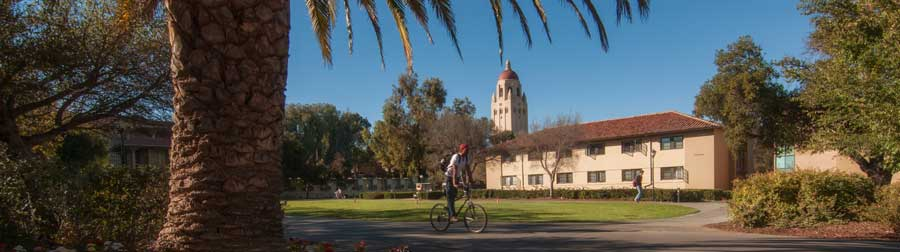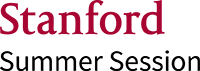Show educations.com as: Mobile

# Summer Course in Computational Engineering: Introduction to Probability and Statistics for Engineers

Stanford University Summer Session
8 weeks
Full time
Summer / Short course
Stanford
English## Summer Course in Computational Engineering: Introduction to Probability and Statistics for Engineers

Random variables, independence, and conditional probability; discrete and continuous distributions, moments, distributions of several random variables.

Topics in mathematical statistics: random sampling, point estimation, confidence intervals, hypothesis testing, non-parametric tests, regression and correlation analyses; applications in engineering, industrial manufacturing, medicine, biology, and other fields.

### Course Details

• Intensive Study: Data Science
• Online Format: Both Synchronous & Asynchronous - This course is taught through a combination of synchronous and asynchronous opportunities.

• Be at least 18 years of age by the start of the study abroad program
• Have graduated from high school or secondary school or equivalent
• Be able to verify fluency in the English language, using one of the methods described in the English Language Proficiency below
• Internet-Based Test of English as a Foreign Language (iBT TOEFL) Minimum score of 95 for the iBT TOEFL, International English Language Testing System: Academic (IELTS: Academic) Minimum overall band score of 7.0, or Cambridge Assessment English Minimum score of 180 on the Cambridge English Advanced, CEFR Level C1.

### Does this course require proof of English proficiency?

The TOEFL® test is accepted by 10,000+ universities and higher education institutes in over 150 countries. Book your test today!## Program content

### Introduction to Probability

• Sample spaces, events, outcomes, axioms of probability
• Counting, permutations, combinations, binomial coefficients
• Probability, conditional probability, Bayes rule, independence
• Random variables, discrete and continuous distributions
• Expectation, moments of a distribution
• Chebyshev’s inequality, characteristic functions, transformations of randomvariables
• Binomial, Poisson, uniform, and normal distributions
• Normal approximation to the binomial distribution, examples
• Exponential distribution, reliability, redundancy
• Distributions of several random variables, moments of joint distributions,independence, covariance, correlation coefficient

### Mathematical Statistics

• Random sampling, parameter estimation, distribution of the sample mean, centrallimit theorem
• Confidence intervals for the mean with known variance, sample variance, biasedand unbiased estimators
• Chi­squared distribution, confidence intervals for the variance, t­distribution,confidence intervals for the mean for small samples
• Maximum -­likelihood estimation, hypothesis testing
• Detection, significance levels, test for the mean of a normal distribution with knownvariance, operating curves
• Test for the mean of a normal distribution with unknown variance, testing aproportion
• Test for the variance of a normal distribution, test for the difference of two means,non­parametric tests: sign test, test for arbitrary trends
• Chi­squared test for the goodness of fit
• Regression analysis
• Correlation analysis

## Tuition fees

The fees for this course is USD 4,700.

## Request info

Want to know more about this program, Summer Course in Computational Engineering: Introduction to Probability and Statistics for Engineers? Fill out the following form and include any questions you have. This information will be sent directly to the school, and a representative will respond to your enquiry.

Date of birth

## VideosStanford University Summer Session invites visiting undergraduate, graduate, and high school students to experience Stanford University during its fourth academic quarter. During the eight-week course, Summer Session students are enrolled in the same courses as matriculated Stanford students, and earn...

## Stanford University Summer Session

94063 Redwood City California
United States of America

Show phone number

Request information

Want to know more about Summer Course in Computational Engineering: Introduction to Probability and Statistics for Engineers? Fill out the following form and we'll pass your details on to a representative from the school, who will respond to your enquiry.

Date of birth
Reviews

Be the first to write a review!## Career test - find your perfect career

Take our free career test that will match you with programs and careers based on your interests and personality.